# Normal algorithm

(diff) ← Older revision | Latest revision (diff) | Newer revision → (diff)

The name attached to algorithms (cf. Algorithm) of a certain precisely characterized type. Together with recursive functions (cf. Recursive function) and Turing machines (cf. Turing machine), normal algorithms have become known as one of the most suitable refinements of the general intuitive idea of an algorithm. The concept of a normal algorithm was developed in 1947 by A.A. Markov in the course of his research on the identity problem for associative systems (see Associative calculus). The detailed definition and general theory of normal algorithms are set forth in  (Chapts. I–V); see also  (Chapts. I–VII).

Every normal algorithmworks in a certain alphabetand gives rise to a well-defined process performed on words (cf. Word). The specification of this alphabet occurs in the definition ofas an obligatory constituent part, and in the situation in question one says ofthat it is a normal algorithm in the alphabet. Every normal algorithm in a fixed alphabetis completely determined by indicating its scheme — an ordered finite list of substitution formulas in. Every such formula is in essence an ordered pair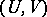of words in. Hereis called the left andthe right part of the formula. Among the formulas of a given scheme some are specially distinguished and are declared to be conclusive. As a rule, in the scheme of a normal algorithm a conclusive formula is written in the formand an inconclusive one in the form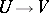.

A normal algorithmin an alphabetis a prescription for constructing, starting from an arbitrary wordin, a sequence of words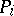according to the following rule. The wordis taken as the initial term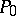of the sequence and the construction process continues. Suppose that for somethe wordhas been constructed and that the process of constructing the sequence in question is not yet complete. If in the scheme ofthere are no formulas with left parts occurring in, then one putsequal to, and the process of constructing the sequence is considered complete. But if in the scheme ofthere are formulas with left parts occurring in, then one takes as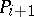the result of substituting the right part of the first of these formulas for the first occurrence of its left part in(cf. Imbedded word); here the process of constructing the sequence is taken to be complete if the substitution formula to be applied at this stage is conclusive and it continues otherwise. If the process of constructing the relevant sequence terminates, then one says that the normal algorithmin question is applicable to the word. The last termof the sequence is the result of applyingtoand is denoted by the symbol. One says thattransformsintoand writes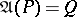. A normal algorithm in any extension ofis said to be a normal algorithm over this alphabet.

There are strong reasons to suppose that the concept of a normal algorithm is an adequate precise version (formalization) of the general idea of an algorithm in an alphabet. More accurately, one may assume that for every algorithmin any alphabetone can construct a normal algorithm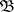overthat transforms an arbitrary wordininto the same result as the original algorithm. This convention is known in the theory of algorithms as the normalization principle. The formalization of the concept of an algorithm on the basis of that of a normal algorithm turns out to be equivalent to other known formalizations (see, for example, ). As a consequence, this normalization principle turns out to be equivalent to the Church thesis, which proposes to assume that the concept of a partial recursive function is an adequate formalization of the non-formal, intuitive, concept of a computable arithmetic function (cf. Computable function).

Originating in the first instance in connection with algebraic problems, normal algorithms have proved to be a convenient working tool in much research requiring a precise notion of an algorithm, especially when the basic objects of study are not of arithmetical nature and allow for an appropriate representation in the form of words in certain alphabets (such as situations in constructive analysis, for example).

How to Cite This Entry:
Normal algorithm. Encyclopedia of Mathematics. URL: http://encyclopediaofmath.org/index.php?title=Normal_algorithm&oldid=18976
This article was adapted from an original article by N.M. Nagornyi (originator), which appeared in Encyclopedia of Mathematics - ISBN 1402006098. See original article